## Summary

This assignment was to add adaptive supersampling/anti-aliasing to the ray tracer. It uses the Suffern supersampling algorithm described in the class lecture.

## Scene Setup

This is the same scene used from Assignment 3. I reused it since it already met the requirements which were "a scene that contains at least two spheres, three non-box triangle meshes and two lights, one of which is white."

## Original 1024 x 1024 image with no anti-aliasing

Rendering took 4 seconds and total primary rays(samples) cast was 1,048,576.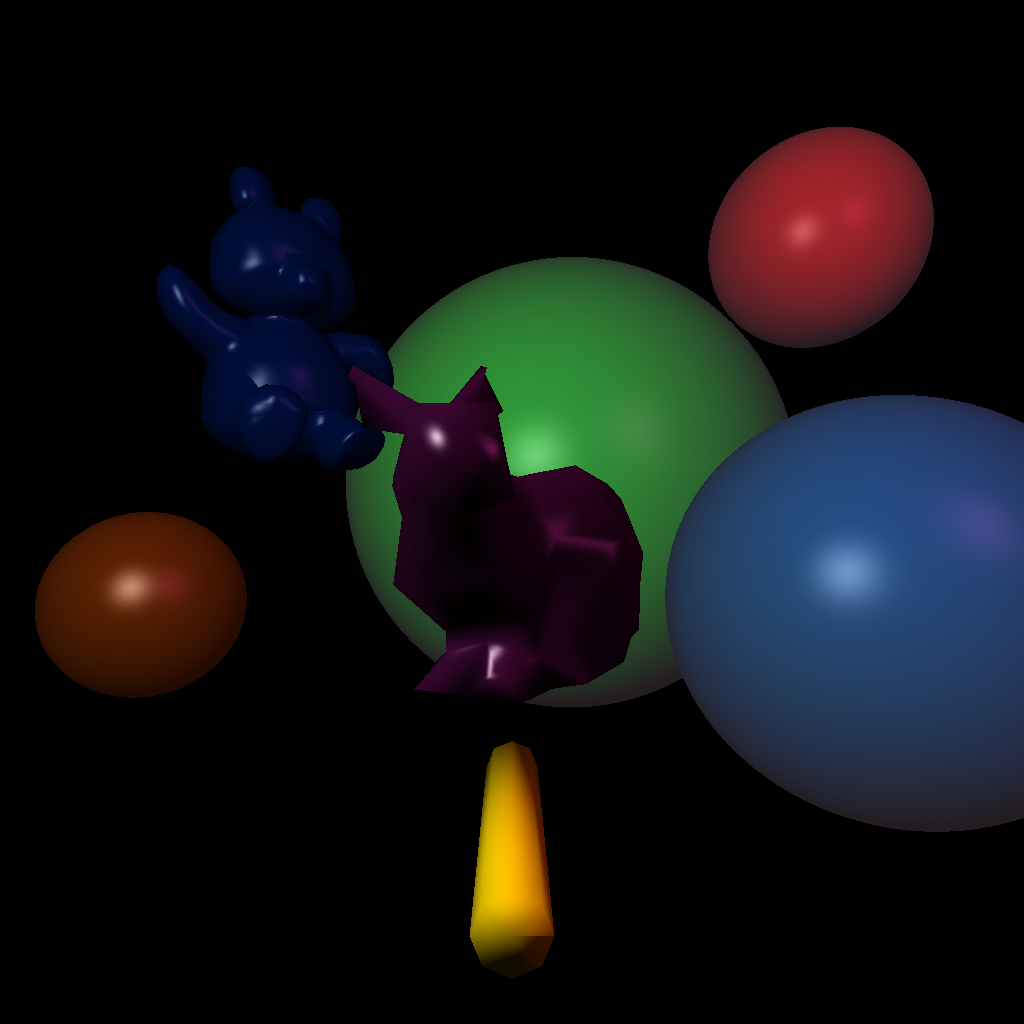## Smoothed 1024 x 1024 image from 2048 x 2048

Rendering took 18 seconds and total primary rays(samples) cast was 4,194,304.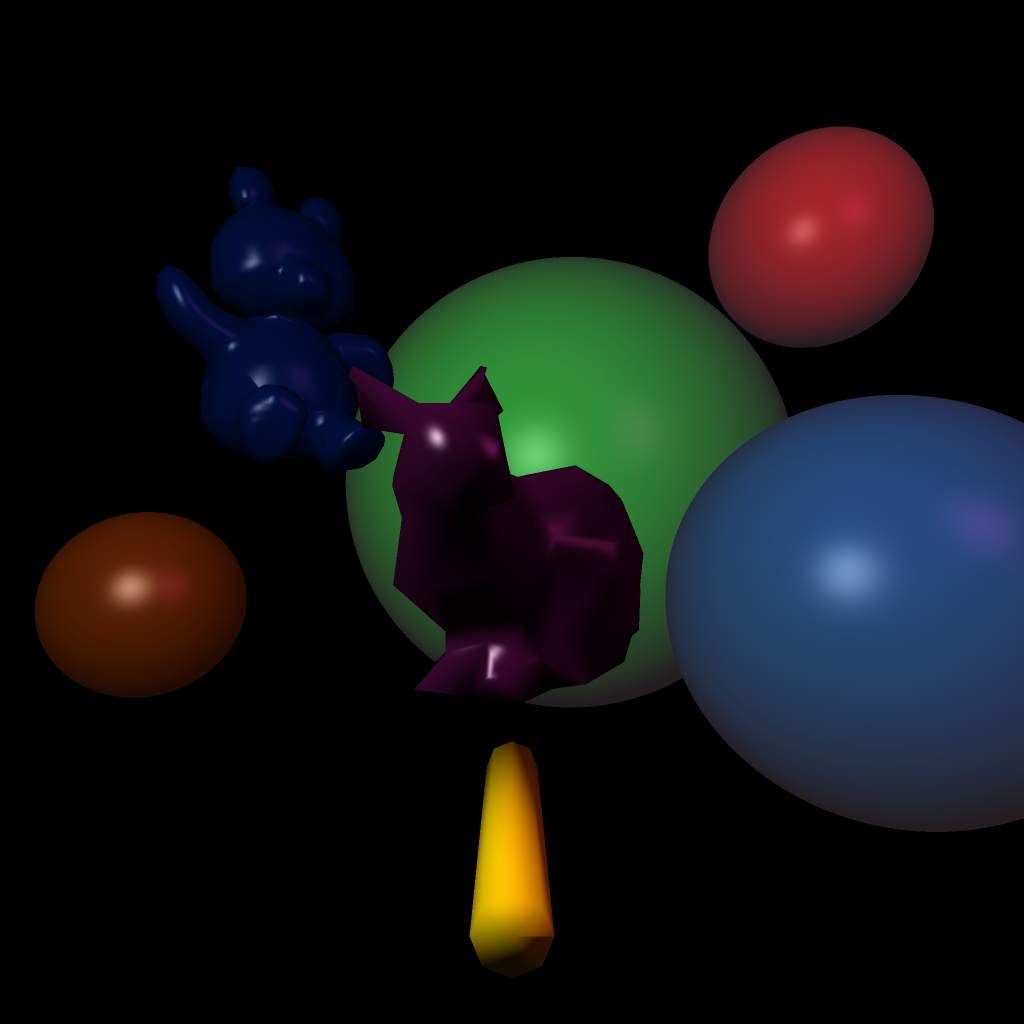## Adaptive supersampling 1024 x 1024 image with tolerance of 10% and two levels of subdivision

Rendering took 6 seconds and total primary rays(samples) cast was 1,132,305.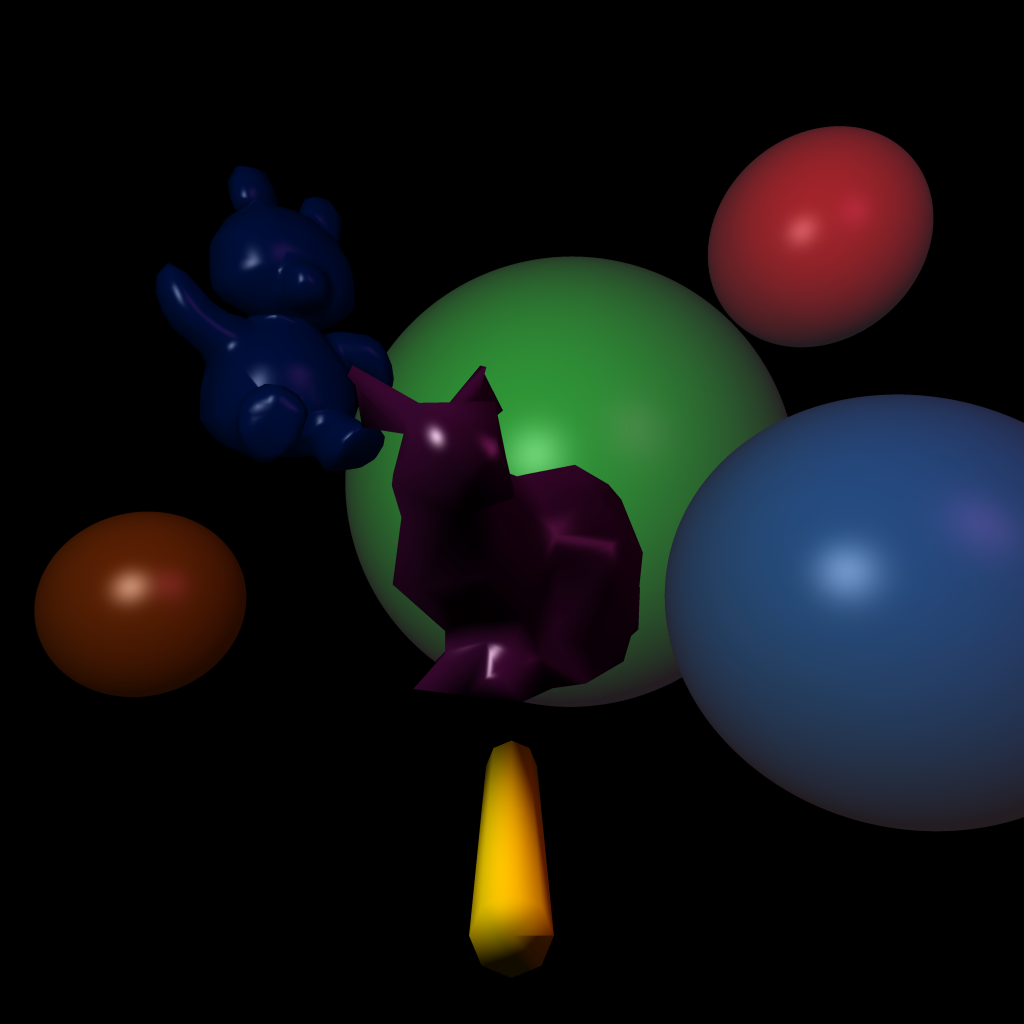## Adaptive supersampling 1024 x 1024 image with tolerance of 10% and two levels of subdivision## Close ups

These images show a section of each of the 3 images above zoomed in 4x original size.

### Original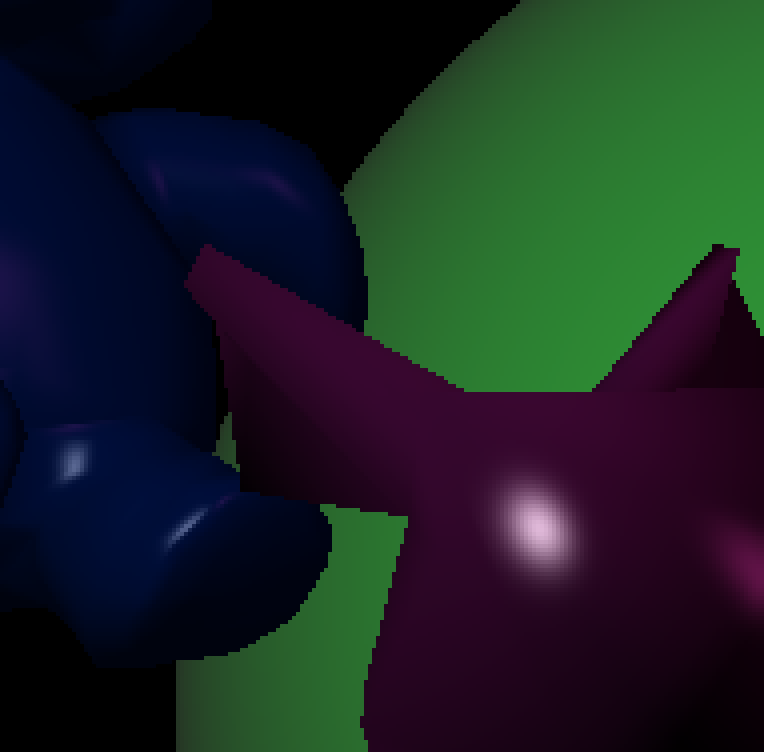### Smoothed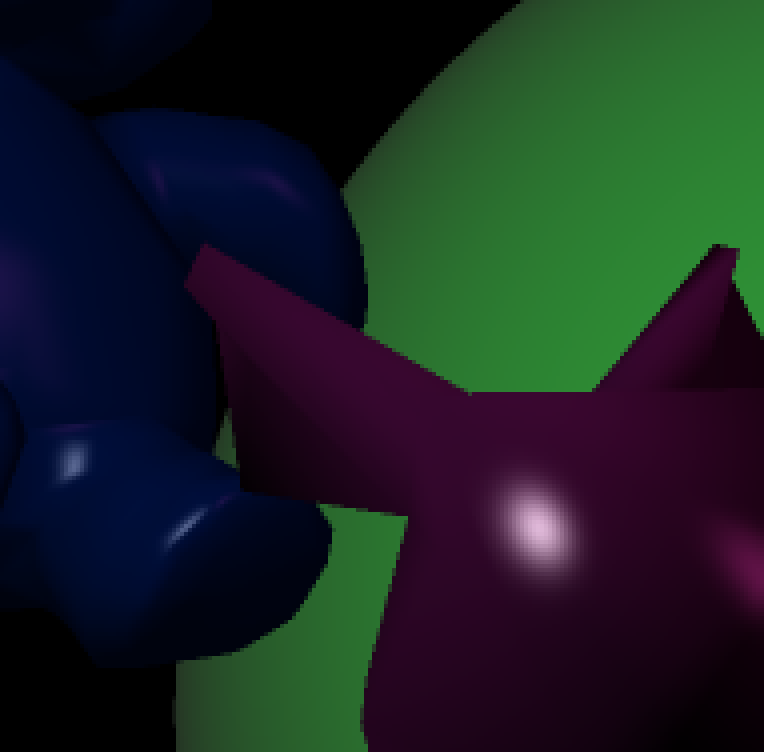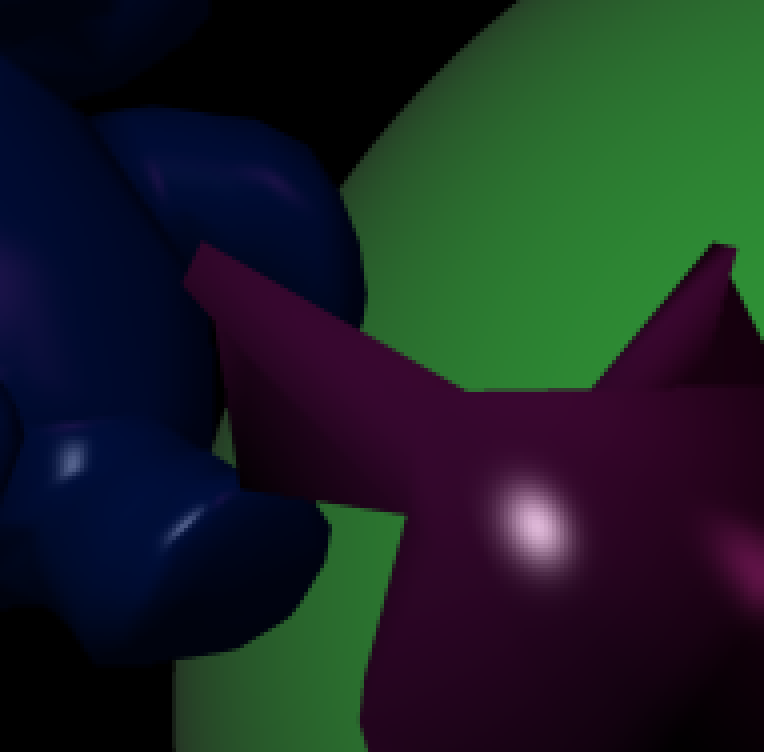### Actual size Original, Smoothed, Adaptive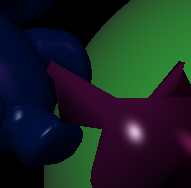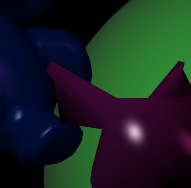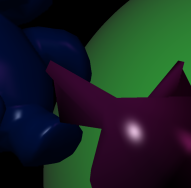## Sample counts images

These images show a monochrome brightness proportionate to the samples used per pixel.

### 5% Tolerance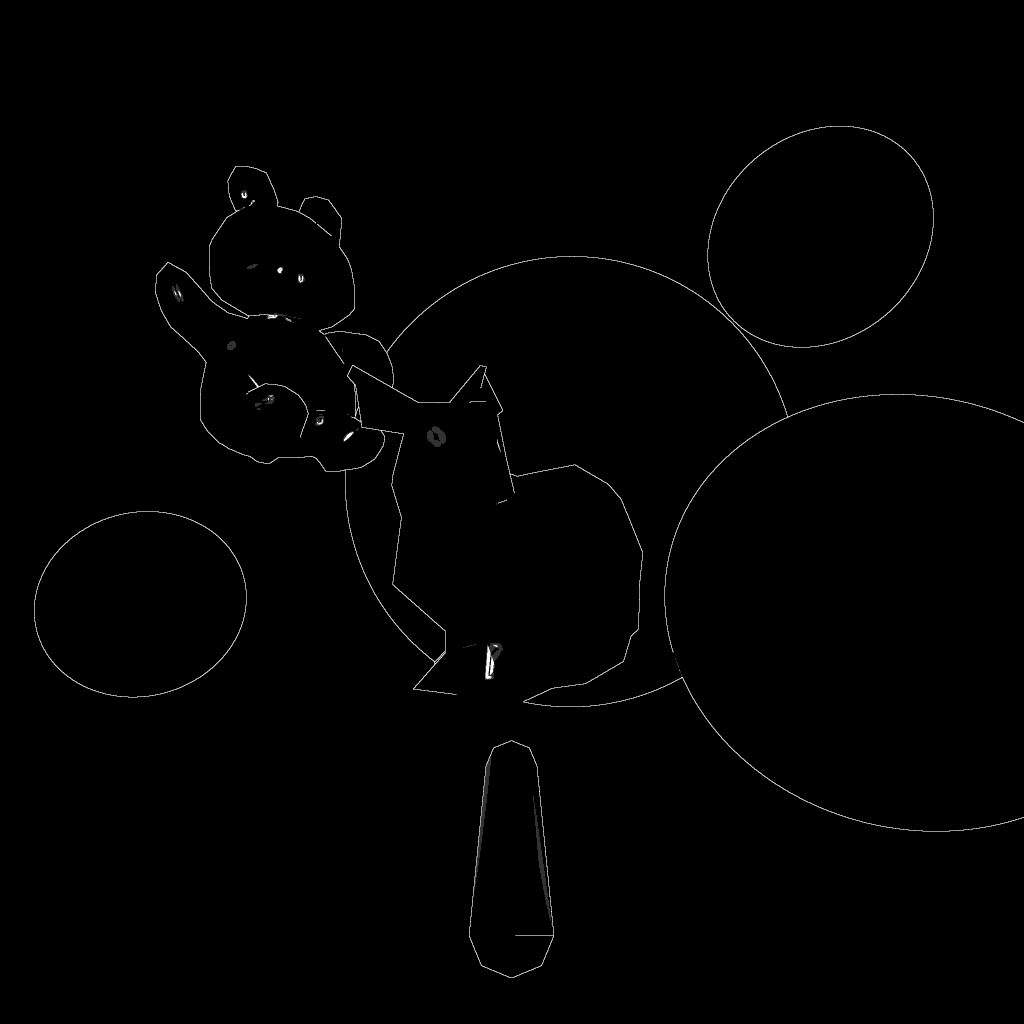### 10% Tolerance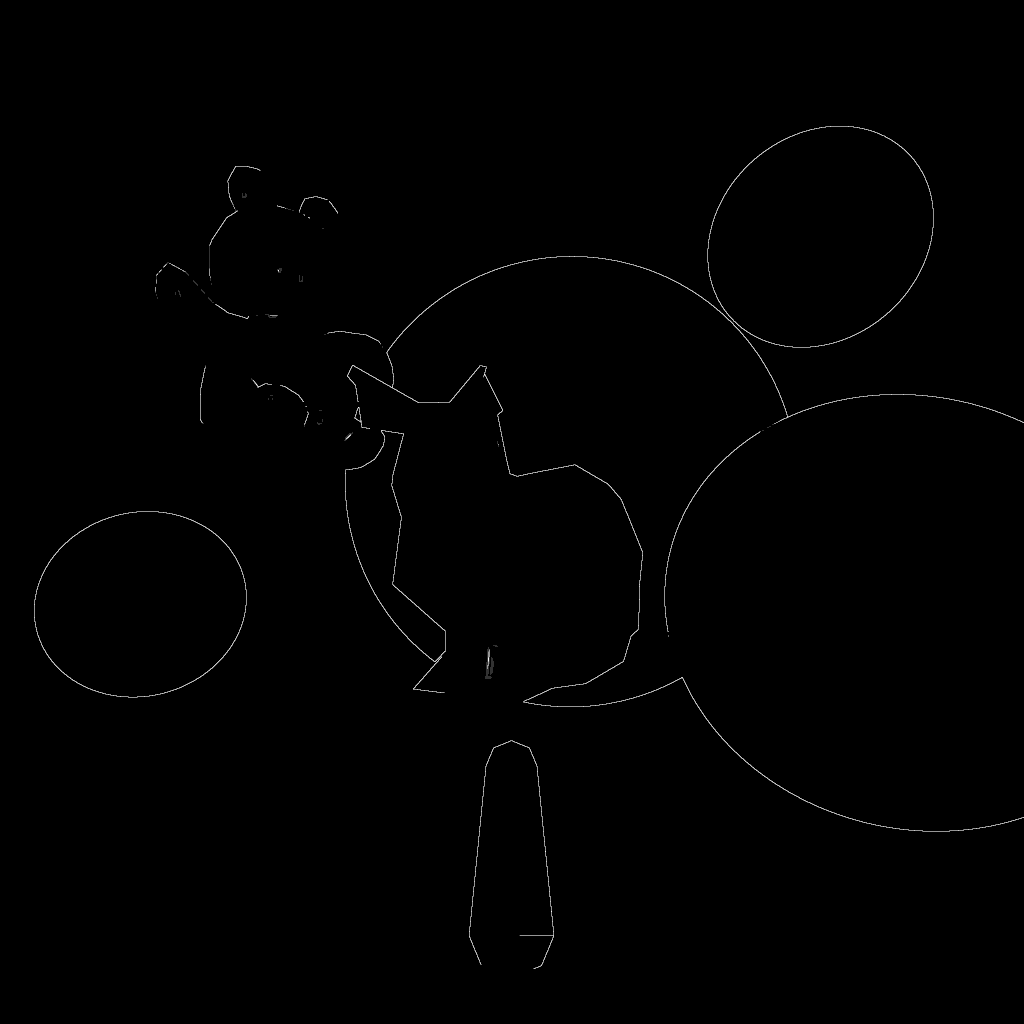### 20% Tolerance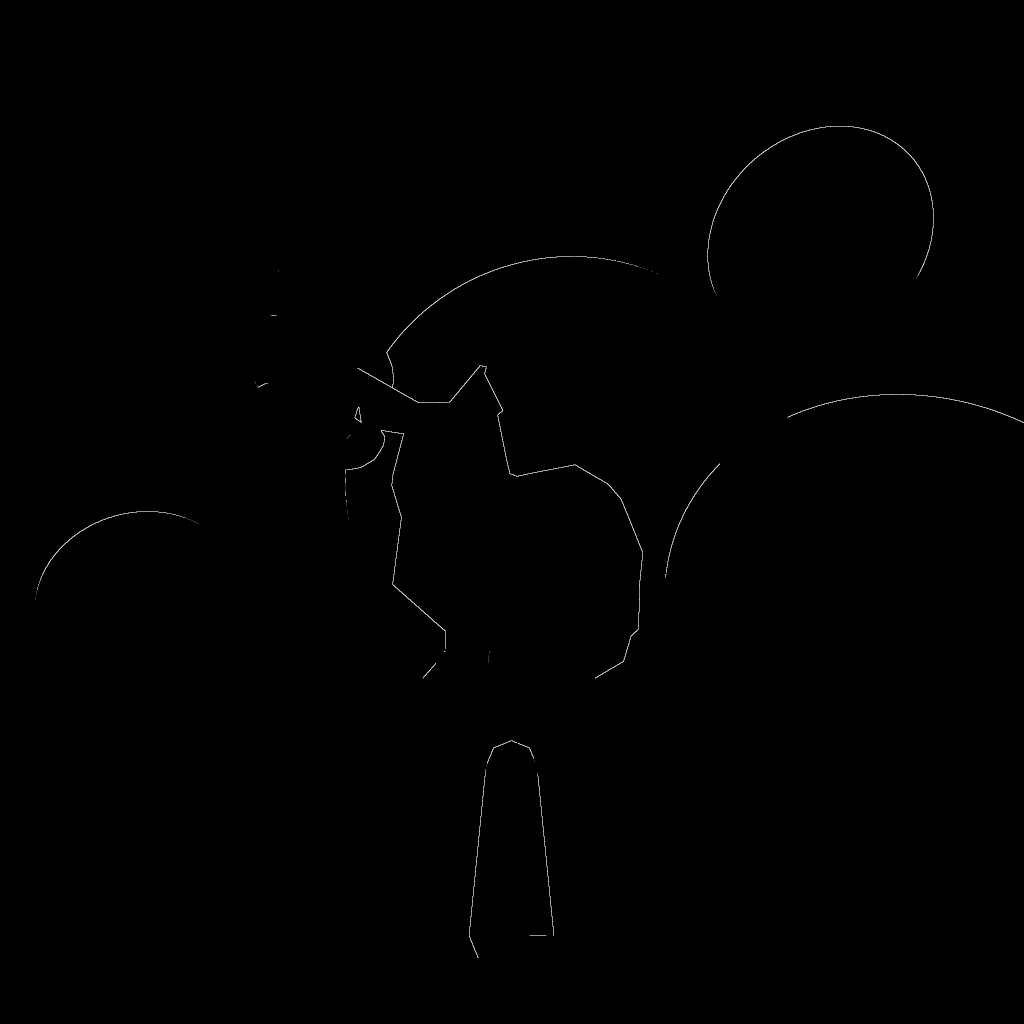## Scene Setup Details

The camera for the scene is setup at (0,0,4) for the eye point, with a view direction of (0,0,-1) and a view up direction of (0, 1,0 ). The view plane distance is 6, and the horizontal camera angle is PI / 6.

The scene has two point light sources: a white light at (-5, 5, 5), and light source with color (0.4, 0.1, 0.3) rgb at position (13, 10, 5);

The scene contains 7 objects: four spheres and three mesh objects including a and a smooth shaded cylinder, a smooth shaded bunny, and a smooth shaded teddy bear.

### Bunny

The bunny is translated along the z axis +2 to move it closer to the camera from the original mesh in "bound-bunny_200.smf". It is also rotated 45 degrees around the Y axis.
• Diffuse surface color of purple (r,g,b) = (0.7, 0.1, 0.6)
• Specular surface color of white (r,g,b) = (1,1,1)
• Ambient surface color of purple (r,g,b) = (0.7, 0.1, 0.6)
• Diffuse coefficient of 0.3
• Specular coefficient of 0.7
• Ambient coefficient of 0.01
• Specular exponent of 30

### First Sphere

The first sphere (on the right) is centered at (8, -2, -10) with a radius of 4.5.
• Diffuse surface color of medium blue (r,g,b) = (3/255, 83/255, 151/255)
• Specular surface color of white (r,g,b) = (1,1,1)
• Ambient surface color of white (r,g,b) = (1,1,1)
• Diffuse coefficient of 0.8.
• Specular coefficient of 0.4
• Ambient coefficient of 0.1
• Specular exponent of 25

### Second Sphere

The second sphere (on the left) is centered at (-8, -2, -10) with a radius of 2.
• Surface color(diffuse and ambient) of orange (r,g,b) = (232/255, 99/255, 10/255)
• Specular surface color of white (r,g,b) = (1,1,1)
• Diffuse coefficient of 0.3.
• Specular coefficient of 0.6
• Ambient coefficient of 0.1
• Specular exponent of 15

### Third Sphere

The third sphere, the large one in the background, is centered at (2, 1, -20) with a radius of 8.
• Surface color(diffuse and ambient) of green (r,g,b) = (20/255, 252/255, 40/255)
• Specular surface color of white (r,g,b) = (1,1,1)
• Diffuse coefficient of 0.8.
• Specular coefficient of 0.4
• Ambient coefficient of 0.1
• Specular exponent of 25

### Fourth Sphere

The fourth sphere, the one to the top right, is centered at (9, 8, -15) with a radius of 3.
• Surface color(diffuse and ambient) of red (r,g,b) = (252/255, 20/255, 20/255)
• Specular surface color of white (r,g,b) = (1,1,1)
• Diffuse coefficient of 0.8.
• Specular coefficient of 0.4
• Ambient coefficient of 0.1
• Specular exponent of 25

The mesh for the teddy bear is translated by (-5, 4, -10), scaled by a factor of 0.15, and rotated around Y axis by PI / 4.

### Teddy Bear

• Surface color (diffuse and ambient) dark blue (r,g,b) = (0, 30/255, 108/255)
• Specular surface color of white (r,g,b) = (1,1,1)
• Diffuse coefficient of 0.5
• Specular coefficient of 0.5
• Ambient coefficient of 0.1
• Specular exponent of 25

### Cylinder

The cylinder is translated along the y axis -2 to move it lower down in the scene.
• Diffuse, specular, and ambient surface color of yellow (r,g,b) = (252/255, 217/255, 0)
• Diffuse coefficient of 0.8
• Specular coefficient of 0.05
• Ambient coefficient of 0.05
• Specular exponent of 2